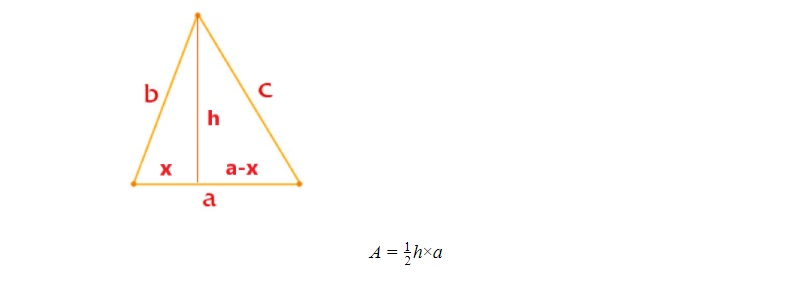# Area of a Triangle: So Many Ways to Find It!Have you ever wondered why the area of any shape is found by using a specific formula and where that formula comes from?

In this blog post we are going to discuss different ways of finding the area of a triangle.

We will go over a number of area of triangle formulas, with the intention to demonstrate how you can derive the formula by yourself having some basic skills of algebra and geometry.

In one of our previous blog posts we have already discussed how to derive the most common area of a triangle formula (click on the image to read the blog post)

Now, let’s look at other formulas and how they are derived.

## The area of a triangle given two sides and the angle between themAs you may already know, in a right triangle, given one of the non-right angles, the relationship between the opposite side and the hypotenuse can be expressed as a sin ratio of that angle.

Let’s construct the height in the above triangle:So, using the common area of a triangle formula, we can create another formula## The area of a triangle using Heron’s formula

This formula was named after Hero of Alexandria, also known as Heron, and is useful when all 3 sides of a triangle are known.which could be easily memorized, but we will do some calculations to produce the formula.

Let’s first draw the height and divide the base side into two:Since we do not know the height, we need to express it in terms of a, b and c.

As we can see, the above triangle is made up of 2 right triangles, so we can use Pythagorean Theorem to express the h.The above method looks a bit complicated, but what is most important here is that we have only used Pythagorean Theorem and some algebraic manipulations to produce the new formula.

## Area of a triangle given 3 heights (advanced topic)

This method of finding the area of a triangle is useful when we know 3 heights of a triangle.The area of the triangle can be expressed by the following:Let’s use the Heron’s formula here:Using Heron’s formula and removing the square root:Let’s simplify:Well, the formula looks complicated, but it has something in common with Heron’s formula.Even the above list of possible formulas is not complete. But most of the formulas are based on the most common area of a triangle formulaWant to improve your math skills and practice more math? Check out IntoMath!

0